## Search This Blog

Simplicity is the ultimate sophistication.” — Leonardo da Vinci
Contact me: sreramk360@gmail.com

## Friday, 20 February 2015

### Learn files programming in C: practical tutorial 3 (Brief introduction to files in C)

"In the Last tutorial, I got a bit carried away. The first question I asked was a bit too much for most C beginners; but if you have a good experience programming in other languages and had shifted to learning C, then I guess that the previous tutorial might have been of at-most use to you in understanding basic C file handling functions." -K Sreram

"To follow this tutorial, you should be familiar with the concepts listed in the tutorial 1 and should have gone through tutorial 2. If you didn't understand the entire program in tutorial 2, don't worry. It will soon make sense as you follow these tutorials.

"To follow this tutorial you should be familiar with the basic file opening and closing operations using fopen, fclose; and should also be familiar with fscanf and fprintf operations to do simple read write operations."

As listed out in tutorial 1, there are a variety of functions for read and write; its better to know all of them; we may choose the one opt for the particular situation we face. Let me give you example programs using those functions and explain their use in detail.

Section 1: using functions fopen, fclose, fgets, fputs
program 1: using functions fopen, fgets, fclose:
/*
* this program example uses fgets function to get an input from a file that already exist
* prototype: char* fgets (char* variable, int size, FILE* fileStream);
*/
#include <stdio.h> // for functions: fopen, fgets, fclose
#include <conio.h> // for function: getch
int main()
{
char text;
FILE* f = fopen("fName.txt", "r"); //opens file named fName.txt, present in the local directory in read mode
if(f==NULL) // checks if the file opened correctly
{
printf("error: unable to open file fName.txt");
getch();
return -1; // returns error signal
}
while(!(fgets(text, sizeof(char)*100, f)==NULL)) //attempts to read data from the file using gets
printf("%s\n", &text); // displays the data
/* This function causes data to be read in blocks of sizeof(char)*100 units each time. Each time the
* characters are read, they are displayed on the application's console output screen. It stops reading
* a line either if it encounters a next line character or the exceeds the size (here its 100)
*/
fclose(f); // clearing all pointers and closing the stream
getch();
return 0;
}

This program just reads data from a file and displays them on the screen. Note that we had given the size of the text variable as a parameter along with the C string as another parameter and the stream to which the data is to be written as another parameter. This size parameter ensures that only a certain number of characters are read from the steam and written to the variable named 'text'; its also to ensure the safety of the function. The at-most number of values the 'text' parameter can hold is of size 'sizeof(char)*100'. If the value entered exceeds the maximum size of the C string, we can expect a run-time error of attempting to access an inaccessible memory location. when we either declare a variable or dynamically allocate one, a specific memory location automatically gets reserved for our program. If we attempt to read from a memory location that's not reserved for our program, then the operating system terminates the program immediately. The fgets function stops reading and returns when it comes across the following characters: '\n', '\0', '\r'. fgets returns the pointer to the string text, in the above case; if the read operation had been a failure, it returns the value NULL. Again, this function is only used for text based read operation.

basic causes for the failure of "fgets" operation (during failure, fgets returns NULL):
1. The file being read is accessed by another application.
2. The end-of-file is reached.
program 2: using functions fopen, fputs, fclose:

/*
* this program example uses fputs function to write to a file
* prototype: int fputs (char* variable, FILE* fileStream);
*/
#include <stdio.h> // for functions: fopen, fgets, fclose
#include <conio.h> // for function: getch
#include <strings.h>
int main()
{
char text;
FILE* f = fopen("fName.txt", "w"); //opens file named fName.txt, present in the local directory in write mode
if(f==NULL) // checks if the file opened correctly
{
printf("error: unable to open file fName.txt");
getch();
return -1; // returns error signal
}
while (1)// starts an infinite loop
{
gets(text); // obtains the text as input
if(strcmp(text, "exit") == NULL) // checks for the string in text: if its "exit" the loop terminates
break;
if(fputs(text, f) == EOF) // writes data to file, and checks if its done right.
{
printf("unable to write to file");
getch();
return -1;
}

}

fclose(f); // clearing all pointers and closing the stream
getch();
return 0;
}

There is one difference between determining end-of-file, while reading data from a file using fgets and writing data do a file using fputs, fgets does not return an integer type signal of the status of reading from the file, instead returns the pointer to the C string to which the read data is stored; In case of failure, a null pointer is returned (i.e., 0). Whereas, fputs returns EOF (or -1) in case of failure and zero in case of success. There is a specific function in C for testing if the file had reached the end-of-file. Its 'int feof(FILE* streamName);' this returns a non zero value when the pointer had reached the end-of-file. This signals the end of file. But if the pointer hadn't reached the file's end, zero is returned.

Using feof when other functions used here accomplishes the same task is not desirable. In this case, the run-time of the program is very less and making slight changes such as adding an feof function to detect the file's end (while reading the data from file) is not going to make any difference. But in real time situations where each function is bound to be called many times before the termination of a particular task or the program, we may avoid calling certain functions often or unnecessary as it could hinder the run-time of the task or the application drastically.

program 3: using functions fopen, fclose, fgets, fputs:
/*
*  program to copy the contents of a text file to another;
*/

#include <stdio.h>
int main()
{
FILE *f_read, *f_write; // a stream for reading and another stream for writing
char readFile,writeFile; // names of files or directories are stored here. Note that
// for windows operating system, the value 260 denotes the maximum
// size of a file name
char data; // a C string used as a temporary buffer to store read data and to write
// it to the destined file.

printf("enter the name of the file to read from (or its directory):"); // get the names or paths of the files
printf("enter the name of the file to write to (or its directory):");
gets(writeFile);

f_write = fopen(writeFile, "w"); // opens the second stream in write mode

while(!(fgets(data, 100, f_read) == NULL)) // gets data until a read error occurs.
fputs(data, f_write); //writes data to stream.

fclose(f_read); // close the streams and clear all memory.
fclose(f_write);
// an alternative to substitute the above two statements could be fcloseall()
return 0;
}

The above code asks for the name/path of the source file to read from and the name/path of the destination file. It then opens both the files and links them to the streams 'f_read' and 'f_write' declared in the main function. Once they are open, the function 'fgets' is made to read data from the file associated with the stream 'f_read' and writes them to the file associated with the stream 'f_write'. This read/write process continues until the 'fgets' function encounters an error. This happens when the file's pointer had reached the file's end.

After the read/write process gets over, both the streams are closed. In this example, both the streams were closed separately, but in situations where there are more than one streams opened and its needed to close them all, then the function 'fcloseall()' can be called. This function closes all the streams other than the basic ones like 'stdin', 'stdout' and 'stderr'.

Section 2: using functions fopen, fclose, fgetc, fputc:
program 1: using functions fopen, fclose, fgetc
/*
* program to count the number of a give character in a particular file using getc
*/

#include <stdio.h>
#include <conio.h>
int main()
{
int count = 0;
char fName, ch, ch2;
FILE *f;
printf("enter the file name:");
gets(fName);
printf("\nenter the character to search:");
scanf("%c", &ch);
f = fopen(fName, "r");
if(f == NULL)
{
printf("error opening file %s", fName);
return -1;
}

while(!((ch2=fgetc(f))==EOF)) //fgetc returns the character that was read from the stream f
if(ch == ch2)   // it returns -1 in case of error. It is because, there isn't any text based
++count;   // character with the value -1.

printf("number of %c's in the file %s is %d", ch, fName,count);
fclose(f);
getch();
return 0;
}

Note that the function fgetc can be interchangeably written as getc. The only difference is, getc is a macro, which is dangerous at some times, and fgetc is a function. Macros are sometimes unsafe. lets say you have a macro defined as '#define foo(a) a*(a+1)/2'. Now, lets pass on an argument, 'b = b+1' to the macro foo. Lets say, foo(b = b +1 ) is written in the calling function. Then, before compilation the macro foo(b = b + 1) is changed to, (b = b + 1)*((b = b + 1)+1)/2. If you watch closely, b is incremented by one twice! this deceives the programmer into believing that b does not increment twice but just increments once, by just glancing at the line 'foo(b = b + 1)'. This makes it more difficult to debug. So its better to use a function rather than a macro. But if you persist on using a macro rather than a function, its advisable to define the macro as an inline statement.

program 2: using functions fopen, fclose, fgetc, fputc:

/*
* program to read text form a text file and convert each sentence's
* starting word's starting letter to upper case. There is a grammatical
* rule in English according to which the sentence's starting word's starting
* letter should be made upper case compulsorily.
*/

#include <stdio.h>
#include <conio.h>
#include <ctype.h>// for isalpha and toupper
int main()
{
FILE *fr, *fw;
char rfName, wfName, data;
int truth = 1; // signals 1 if the next character read should be converted to upper-case

printf("enter the name of the file to read from (or the directory):");
gets(rfName);
printf("\nenter the name of the file to write to (or its directory):");
gets(wfName);

fr = fopen(rfName, "r");
if(fr == NULL)
{
printf("\nunable to open file %s", rfName);
return -1;
}

fw = fopen(wfName, "w");
if(fw == NULL)
{
printf("\nunable to open file %s", wfName);
return -1;
}

while(!feof(fr))
{
data = fgetc(fr);
if(data == EOF)
{
return -1;
}
else if(data == '.' || data == '!' || data == '?')
truth = 1;
else if(isalpha(data) && truth)
{
data = toupper(data);
truth = 0;
}
if(fputc(data, fw) == EOF) // fputc returns EOF if there was a error reading and sets stderr
{
printf("error writing to file");
return -1;
}
}
fclose(fw);
fclose(fw);
getch();
return 0;
}

This program helps the user convert the starting letter of each sentence to capital, is the user had forgotten to do so. The program reads a character from the file to be read from and checks if its '.' or '?' or '!'. If the character read was either of these, then the value 'truth' is set to one. Now, if the next character read was an alphabet, then it gets converted to upper case before being written to the other file, which is opened in write mode. Then, the value to 'truth' is set to zero. While the value of truth is set to zero, then the conversion from upper case to lower case does not take place.

copyright (c) 2015 K Sreram. You may not distribute this article without the concerned permission from K Sreram.

## Monday, 16 February 2015

### a short program for the inverse of a 3X3 matrix in C

A short program for the inverse of a 3X3 matrix in C

The following is a short program for inverse of a $3 \times 3$ ordered matrix. It uses the mathematical formula $A^{-1} = \frac {adj(A)}{|A|}$. There are three functions, one for obtaining the minors, the other for finding the determinant and the other for finding the adjoin. The part of dividing the adjoin by the determinant is computed in the main function and the inverse matrix is displayed as the result.

/**
* a short program to find the inverse of a matrix
* By K Sreram
*/
#include <stdio.h>
#include <conio.h>
/*************************************
*  finds the determinant of each of the minors
**************************************
*/
float detMinor(int y, int x, float matrix)
{/// finds the determinant of the minors
int i,j;
float mt;
for(i=0; i<3; i++)
for(j=0; j<3; j++)
{
if(i==y || j==x)
continue;
mt[i-(i>y)][j-(j>x)]=matrix[i][j];
}
return (mt*mt-mt*mt);
}
/**************************************
*  finds the determinant of a $3\times3$ matrix
***************************************
*/
float determinent3x3(float matrix)
{/// finds the determinant of the 3x3 matrix
return (detMinor(0,0,matrix)*matrix-
detMinor(0,1,matrix)*matrix+detMinor(0,2,matrix)*matrix);
}
/*************************************
*   finds the adjoin of the matrix
**************************************
*/
{/// finds the adjoin of the matrix m_from and stores it in m_to
int i,j,a=1;
for(i=0; i<3; i++)
for(j=0; j<3; j++)
{
if((i+j)%2==0)
a=1;
else
a=-1;
m_to[j][i]=a*detMinor(i,j,m_from);
}
}
int main()
{
int i,j;
float matrix,m_inv,d;
printf("enter the 3x3 matrix:");   /// ask for the matrix as an user input
for(i=0; i<3; i++)
for(j=0; j<3; j++)
scanf("%f",&matrix[i][j]);    /// get the input
d=determinent3x3(matrix); /// find the determinant and store it in the variable d
if(d==0)   /// inverse does not exist if the determinant is zero
{
printf("inverse does not exist as determinant is zero");
getch();
return 0;
}
for(i=0; i<3; i++)
for(j=0; j<3; j++) ///divides each element by d
{
m_inv[i][j]= m_inv[i][j]/d;
} /// by now the major computation process is over
for(i=0; i<3; i++)
{
for(j=0; j<3; j++)/// displays the inverse matrix
{
printf("\t%f",m_inv[i][j] );
}
printf("\n");
}
getch();
return 0;
}

# The mega confusion about infinity clarified!

By K Sreram
An answer to my friend’s question posted in that group.
The most “complicated number” in mathematics is infinity. It’s not because it’s extremely large or indeterminable or something, it’s because it inherits unique properties which other numbers in the set of real numbers don’t possess. And your question (refer to the group for the asked question) is about infinity that is not actually infinity!!! How am I saying that? It’s simple: you have been talking about numbers that nearly approach infinity (which anyone might say is logically impossible. Imagine a number as large as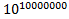. There is always a number greater than that number (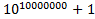). But we can say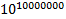is almost equal to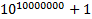because, beside such a large number 1 has no value! So we can never make a number approach infinity and we always say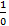as purely undefined or it does not refer to anything at all.
But that’s not the same infinity (it is not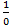) you have been talking about in that question. Before confusing you further, let me put forward the actual definition of the infinity you have been talking about: a number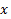that is always greater than the limiting number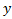. Note that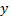is a perfect number (i.e., belongs to the set of real numbers). So we can write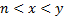and let’s assume that the value of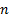andbecome extremely large but not infinity, then we have ourvalue which we are free to consider as infinity.
Note: in differential calculus and limits and any other subject involving these concepts, do not talk about the actual infinity but an extremely large and indeterminable number which exceeds the maximum limit of our measuring scale and which our measuring scale marks as: “unmeasurable large!”.
We can say that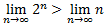. Note that here we are not writing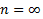but instead we are writing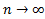. The later expression is different from the former expression. The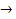stands for “approaches” and does not stand for “is equal”.
A little math: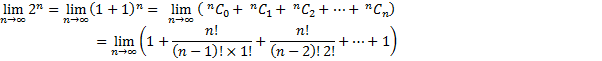Or we can write,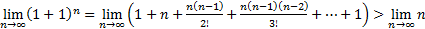.
So we have one infinity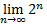greater than the other infinity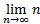.

Update:
There is one more thing I would like to add. There is an answer among people that fits their imagination and quenches their curiosity because they refuse to think further. They believe that even the absolute infinity can be subjected to be compared. But that’s not the case. Many of us say that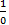is infinity, but I wish to sayis plainly undefined if the zero in the denominator is an absolute zero and not infinity! Let’s say that there is an equation of some sort. Then we would have the LHS and the RHS with either an equal to sign or other arithmetic comparison symbol separating them. But what is the equation’s solution? It’s simple: on trying out all the possible numerical or expression substation to the present unknown symbol in the equation, the one(s) that satisfy the equation are chosen as the solution or the solution set. Remember the details of the order by which we substitute the symbol with the possible expression or the numerical value is not present in the equation. We may substitute random values until we get the correct solution. What does all this have to do with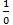? Let me tell you: if the zero in the denominator is absolute zero, then there is no tendency for the zero to increase or decrease further (either go to the negative axis or the positive axis). Let’s write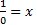or we get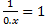. Dose this even make sense? The solution for this equation is for some value of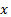the value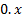becomes one. How will this zero become one if it doesn’t have the tendency to move towards a value? Zero is nothing; it doesn’t have any value at all. How many ever times you add zero, it cannot become something because it doesn’t have the tendency to become something. Note that if there is a specific order by which we substitute values towe might be able to say some answers. If the order is increasing (that is first we substitute 1 and then 2 and then 3 and so on) then our answer is infinity; and that is exactly what is represented by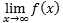. It says thatstarts small and approaches infinity.

One more interesting fact about zero is that, it’s the origin of every dimension known. Let’s consider a one dimensional space coordinate. It has the zero in its center separating the negative and the positive regions. Now imagine another axis; this would make it 2 dimensional space. Again, zero is the meeting point for both the axis. Let’s now extend our imagination to a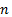dimensional space. Zero, is now the meeting point of all thedimensions! So now if we are to multiply that zero by scalar quantity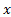and if we are to say that the value of the product is non zero, then out of thesedimensions through which dimension should this product fall under? Note that zero is not a non-vector; it’s a vector. It’s a vector having all directions to move to. It’s like a bridge between higher dimensions.
To put it shortly,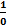has no meaning.

Summary:
The infinity used in many calculations that involve is not actual infinity but an expression that gives a number that can be treated as infinity while adding, subtracting with a finite value or while just writing down the result, but is treated as any other number while doing operations like multiplication, division, either with infinite numbers or finite numbers. In the given example, there are more number of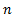’s in the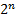expansion than that singlein the linear limit expression.